Electron. J. Diff. Eqns., Vol. 2007(2007), No. 128, pp. 1-18.

### On the wave equations with memory in noncylindrical domains Mauro L. Santos

Abstract:
In this paper we prove the exponential and polynomial decays rates in the case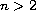, as time approaches infinity of regular solutions of the wave equations with memory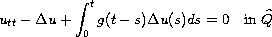where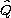is a non cylindrical domains of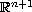,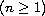. We show that the dissipation produced by memory effect is strong enough to produce exponential decay of solution provided the relaxation functionalso decays exponentially. When the relaxation function decay polynomially, we show that the solution decays polynomially with the same rate. For this we introduced a new multiplier that makes an important role in the obtaining of the exponential and polynomial decays of the energy of the system. Existence, uniqueness and regularity of solutions for any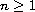are investigated. The obtained result extends known results from cylindrical to non-cylindrical domains.

Submitted March 8, 2007. Published October 2, 2007.
Math Subject Classifications: 35K55, 35F30, 34B15.
Key Words: Wave equation; noncylindrical domain; memory dissipation.

Show me the PDF file (298 KB), TEX file, and other files for this article.Mauro de Lima Santos Faculdade de Matemática, Universidade Federal do Pará Campus Universitario do Guamá, Rua Augusto Corrêa 01, Cep 66075-110, Pará, Brasil email: ls@ufpa.br